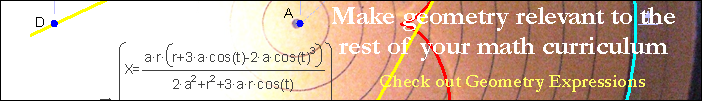AnglesAngle of ElevationThe angle of elevation is the angle formed by the line of sight and the horizontal for an object above the horizontal.Angle of DepressionThe angle of depression is the angle formed by the line of sight and the horizontal for an object below the horizontal.Supplementary AnglesSupplementary angles are angles whose sum equals 180 degrees.Straight AngleA straight angle is any angle equal to 180 degrees or π radians.Corresponding AnglesCorresponding angles are two angles in matching corners, when two lines are cut by a third (the transversal). If the two lines are parallel, then the corresponding angles are congruent.Alternate Interior AnglesWhen two lines are cut by a third line (the transversal), two angles that are on the inside of the first two lines and on opposite sides of the transversal are alternate interior angles. If the two lines are parallel, then the angles are congruent.Vertical AnglesVertical angles share the same vertex and are bounded by the same pair of lines but are opposite to each other.Alternate Exterior AnglesWhen two lines are cut by a third line (the transversal), two angles that are on the outside of the first two lines and on opposite sides of the transversal are alternate exterior angles. If the two lines are parallel, then the angles are congruent.Central AngleA central angle is an angle whose vertex is the center of a circle.Inscribed AngleAn inscribed angle has a vertex and two endpoints on a circle. If two inscribed angles subtend the same arc, then the angles are congruent.Central and Inscribed Angle RelationshipIf an inscribed angle and a central angle subtend the same arc, then the central angle is twice the inscribed angle.Complementary AnglesComplementary Angles are angles whose sum is 90 degrees.Parallel line transversalShows equivalent angles in a parallel line transversal